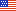An official website of the United States governmentOfficial websites use .gov
A .gov website belongs to an official government organization in the United States.Secure .gov websites use HTTPS
A lock ( ) or https:// means you’ve safely connected to the .gov website. Share sensitive information only on official, secure websites.

# How to Compute Rates of Pay

## Fact Sheet: How to Compute Rates of Pay

### Background

Hourly and biweekly rates for General Schedule employees are computed under 5 U.S.C. 5504. Premium pay is computed under 5 U.S.C. chapter 55, subchapter V, and 5 CFR part 550, subpart A. The rates are computed based on an employee’s annual rate of basic pay, including any applicable special rate supplement or locality payment. (Note: Overtime pay for nonexempt employees is computed under the Fair Labor Standards Act, subject to some special rules for Federal employees. See 5 CFR part 551. In addition, firefighters covered by 5 U.S.C. 5545b are subject to special rules for computing hourly rates and overtime pay. See 5 CFR part 550, subpart M.)

### Rules for Rounding

Rates must be rounded to the nearest cent, counting one-half cent and over as the next higher cent (e.g., round \$18.845 to \$18.85).

### Procedure

Follow the rules below to compute rates of pay. (Note: The examples below are based on annual rates that have been increased by a locality pay percentage of 32.49%. See Salary Table 2023-DCB.)

1. Hourly Rate.

Divide annual rate of basic pay by 2,087 hours.
GS-9, step 1 = \$ 64,957.
\$ 64,957 / 2,087 hours = \$ 31.12.

2. Biweekly Rate.

Multiply hourly rate of basic pay by 80 hours.
\$ 31.12 x 80 hours = \$ 2,489.60.

3. Title 5 Overtime Hourly Rate
1. if employee's rate of basic pay is less than rate of basic pay for GS-10, step 1

Multiply hourly rate of basic pay by 1.5.
\$ 31.12 x 1.5 = \$ 46.68.

2. if employee's rate of basic pay is more than rate of basic pay for GS-10, step 1

Use the greater of (1) the GS-10, step 1, hourly rate of basic pay multiplied by 1.5 OR (2) the employee’s hourly rate of basic pay. (5 CFR 550.113)

GS-10, step 1 = \$ 71,531.
\$ 71,531 / 2,087 hours = \$ 34.27.
\$ 34.27 x 1.5 = \$ 51.41.

GS-14, step 1 = \$ 132,368.
\$ 132,368 / 2,087 hours = \$ 63.43.

In this example, the employee’s hourly rate of basic pay (\$63.43) is greater than the GS-10, step 1, hourly rate of basic pay multiplied by 1.5 (\$51.41). Therefore, the employee’s title 5 overtime hourly rate is equal to his or her hourly rate of basic pay.

4. Night Pay.

Multiply hourly rate of basic pay by 10%. (5 CFR 550.121)
GS-9, step 1 = \$ 64,957.
\$ 64,957 / 2,087 hours = \$ 31.12.
\$ 31.12 x 0.10 = \$ 3.11.